# Video Tutorial: Self-Organizing Maps in MATLAB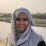Published

on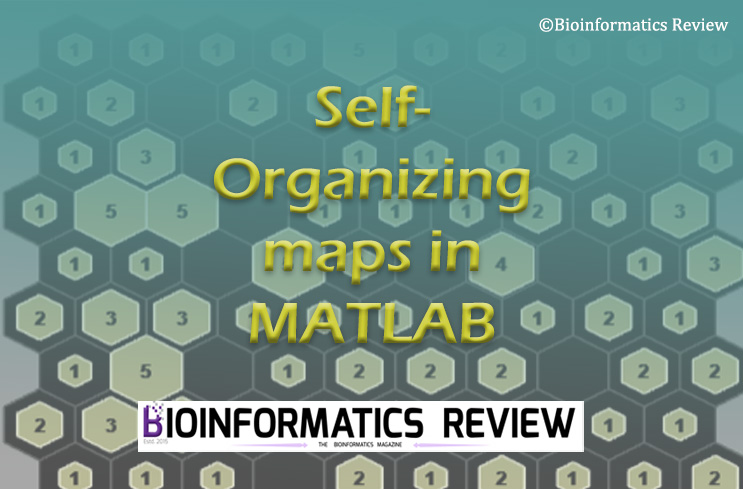Here is a video tutorial for performing self-organizing maps in MATLAB .

#### References

1. MATLAB. (2022). version 7.10.0 (R2022b). Natick, Massachusetts: The MathWorks Inc.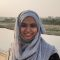Dr. Muniba is a Bioinformatician based in New Delhi, India. She has completed her PhD in Bioinformatics from South China University of Technology, Guangzhou, China. She has cutting edge knowledge of bioinformatics tools, algorithms, and drug designing. When she is not reading she is found enjoying with the family. Know more about Muniba

# How to preprocess data for clustering in MATLAB?Published

on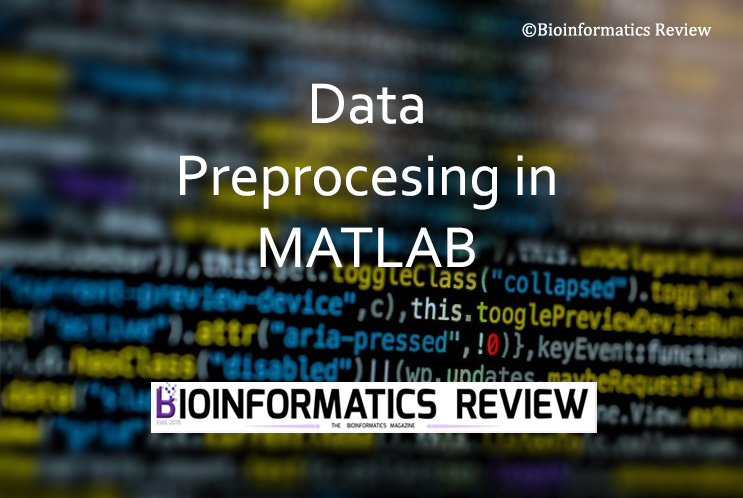Data preprocessing is a foremost and essential step in clustering based on machine learning methods. It removes noise and provides better results. In this article, we are going to discuss the steps involved in data preprocessing using MATLAB . (more…)

# How to define features/predictors of user dataset for SOM analysis in MATLAB?Published

on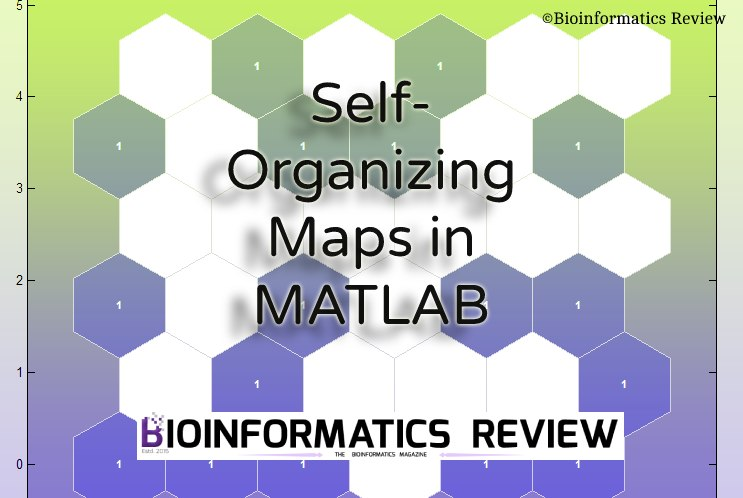Most of the examples that demonstrate the SOM analysis use the default Iris dataset. It is also mentioned in the SOM example of MATLAB that for the user dataset, the predictors need to be defined . But it is not quite clear how to define the features or predictors of our dataset. In this article, we will learn how to define predictors for user datasets. (more…)

# How to get input values from SOM sample hits plot in Matlab?Published

on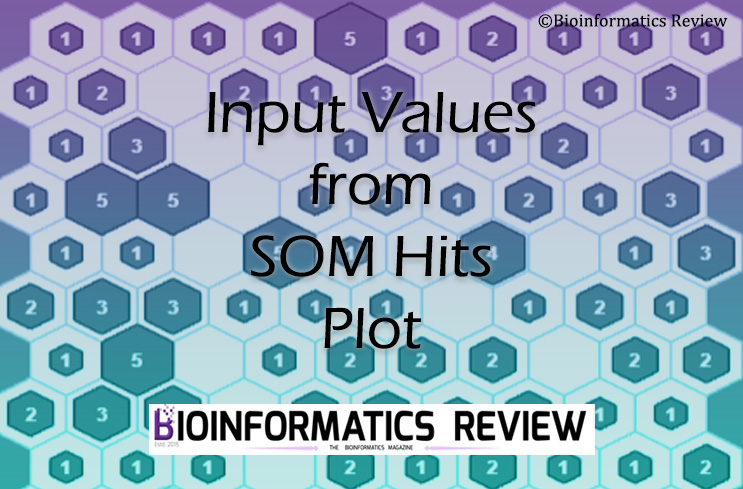The self-organizing map (SOM) sample hits plot in the Matlab  shows the number of inputs in the neurons but one would be interested in knowing what these input values are. In this article, we are going to get the input values from the neurons. (more…)